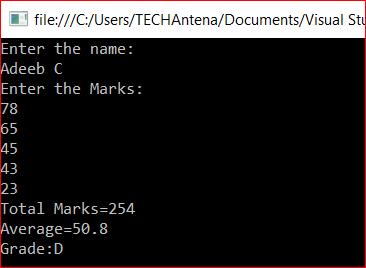# VB.NET Console Application to Calculate Grades From Given Marks

December 12, 2016Here is a console application in VB.NET to calculate the grades from given marks of a student.

Aim:

Create a VB.NET console application to read the name and marks of five subjects of a student. And to find the total, average marks, and to calculate grade of the student as follows.

• Average mark > 90 then Grade = A
• Average mark > 80 then Grade = B
• Average mark > 60 then Grade = C
• Average mark > 40 then Grade = D
• Average mark < 40 then Grade = E

Program Code:

```Module Module1
Sub Main()
Dim m1, m2, m3, m4, m5, total As Integer
Dim name As String
Dim avg As Double
Console.WriteLine("Enter the name:")
Console.WriteLine("Enter the Marks:")
total = m1 + m2 + m3 + m4 + m5
avg = total / 5
Console.WriteLine("Total Marks=" & total)
Console.WriteLine("Average=" & avg)

'divide avg by 10 to make the calculation easier
avg = avg / 10

If (avg >= 9) Then
ElseIf (avg >= 8) Then
ElseIf (avg >= 6) Then
ElseIf (avg >= 4) Then
Else
End If

End Sub
End Module```

Output:

```Enter the name:
Enter the Marks:
78
65
45
43
23
Total Marks:254
Average:50.8